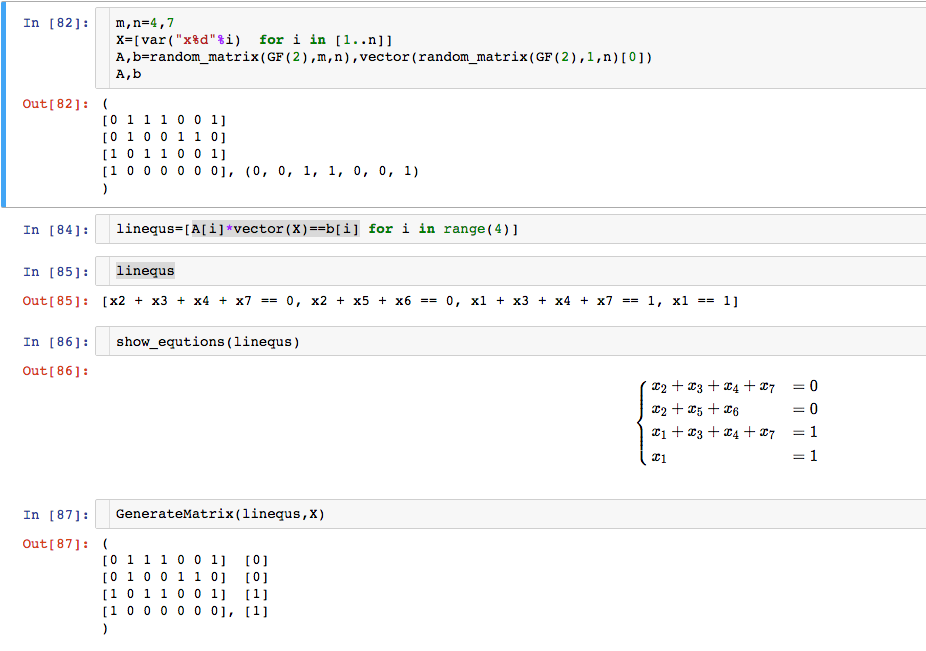# Turning system of linear equations into a matrixAnonymous

I have a very long list of equations (10^3 order of magnitude), but all linear. There are around 10^2 variables.

I would like to solve these equations in $F_2$, that is, in the field of 2 elements. Questions:

1. Is there a handy-dandy "solve" command in Sage that allows me to list these equations, specify the field in which I want these equations to be interpreted, and Sage returns an answer?

2. Or, is there a handy-dandy way I can input a list of linear equations, and Sage automatically returns a matrix? So long as I can read off which entries/columns of the matrix correspond to which variables in my original equations, I'm happy to then use commands taking matrices as inputs, and use the answer to the question "Solve large system of linear equations over GF(2)" (I would put the link in, but I don't have the karma).

edit retag close merge delete

Sort by » oldest newest most voted

I give an answer for question 2. See the following :The definition of GenerateMatrix is:

def GenerateMatrix(equsys, vars):
A=matrix([[equ.lhs().coefficient(v) for v in vars] for equ in equsys])
b=matrix([[equ.rhs()] for equ in equsys])
return (A,b)

more